## Introduction

Diabetes mellitus is a metabolic disorder characterized by a fluctuation in the blood glucose level outside the normal range as a result of the underproduction (type 1) or underutilization (type 2) of the hormone insulin. Diabetes is a complex disease that can potentially affect every body organ through complications such as blindness, kidney failure, heart failure and nerve degeneration1,2,3,4,5. The World Health Organization (WHO) estimated the number of persons with diabetes worldwide to be approximately 347 million in 2013 and this number is increasing at a tremendous rate. Therefore, the control of diabetes still remains a great challenge. However, one study6 previously demonstrated that a step-wise progression in these complications occurs when the blood glucose level increases from the normal fasting plasma glucose level of 0.89 mg/mL to levels exceeding 3.5 mg/mL. Therefore, these complications can be prevented by using a biosensor to closely and accurately monitor the blood glucose level7. When variations in blood glucose level are detected, they can be controlled by appropriate measures, such as diet therapy, exercise, insulin injections and/or oral drugs.

To prevent life-threatening events and the debilitating complications associated with diabetes, researchers into developing glucose biosensors is ongoing. A number of glucose biosensors with various transduction techniques have been reported, including electrochemical, optical and electromagnetic spectroscopy biosensors. Among these types, electrochemical biosensors are the most widely accepted for the sensitive detection of glucose. Non-enzymatic8,9,10,11,12,13 electrochemical glucose sensors are used to overcome the degradation caused by the use of mediators in enzymatic14,15 glucose sensors. However, interference from a co-substrate and increased sensor response time due to the use of outer members degrades the performance of these sensors. Optical sensors are highly specific to glucose. Specifically, Raman spectroscopy exhibits sharper peaks and less overlap and avoids interference from luminescence and fluorescence16,17. However, this spectroscopy method requires longer stabilization times and is affected by the tissue density, tissue thickness and hematocrit. Recently, radiofrequency (RF)-based label-free biosensors for use in applications, such as the detection of stress biomarkers18, biomolecular binding19,20, human cell dielectric spectroscopy21 and glucose detection22,23,24,25,26 have been reported. Glucose sensors based on this technique quantitatively assess glucose levels by observing the level of electromagnetic coupling, which depends on glucose permittivity. This permittivity, in turn, depends on the local glucose concentration. These biosensors, also called third-generation glucose sensors, provide the label-free detection of glucose and exhibit a very short assay time. However, this technology is still plagued by a variety of issues that affect its accuracy and sensitivity.

The objective of this study was to develop a miniaturized RF resonator-based reusable biosensor for the mediator-free detection of glucose in deionized water-glucose solution and human serum with high sensitivity. An interdigital capacitor (IDC) was embedded internally between the two divisions of a spiral inductor to generate a micro-sized resonator with a center frequency suitable for the sensitive detection of glucose. In addition, the inductor coils were intertwined to enhance the mutual inductance, thereby minimizing the loss of the signal transmission. To study the resonator's potential applications in glucose sensing and diabetes monitoring, it was used to detect glucose in a glucose-water solution and human serum. Glucose samples (5 μL) of varying concentrations were dropped on the sensing region of the resonator and the S-parameters were measured. The stabilized measurement results were obtained approximately 2 sec after the samples were dropped on the resonator. The experimental results indicate that the shift in the center frequency of the resonator was clearly detected by a sharp S11 for varying glucose concentrations, which verified that the glucose level could be detected with high sensitivity. In addition, the variations in the S11 magnitude at the center frequency and S21 at the transmission zero were used to enhance the detection accuracy. Moreover, a number of parameters, such as the propagation constant (γ), impedance (Z), resistance (R), inductance (L), conductance (G) and capacitance (C) were estimated from the measured S-parameters to support the multi-dimensional detection of glucose. The resonator, when flushed after taking the measurements, exhibited original resonating characteristics prior to the measurements. This finding suggests that the device can be reused to detect glucose. Atomic force microscopy (AFM) was also used to study the surface roughness of the resonator for various conditions to verify the reusability of the device.

## Results

### Frequency range of the RF biosensing resonator

An IDC was integrated between the two divisions of a square-shaped spiral inductor with rounded corners to generate a compact RF resonator, as illustrated in Fig. 1 (a) and (d). To minimize the loss of signal transmission along the inductor, its turns were intertwined to utilize the enhanced mutual inductance using air-bridge structures, whose enlarged images are depicted in Fig. 1(e) and (f). The center frequency of the proposed resonator can be expressed as fo = 1/2π(LC)1/2, where L and C can be estimated as outlined in previous studies27,28. The dimensions of the resonator were optimized to resonate at a center frequency of 2.246 GHz. This frequency is suitable for the sensitive detection of glucose because of the nature of the interactions among the glucose, constituents and field frequency, in which water interactions with the field significantly dominate all other constituents at microwave frequencies between 0.915 and 2.45 GHz29,30. Fig. 1 (a) also illustrates the equivalent resonator circuit in terms of R, which accounts for the resistive loss of the spiral inductor of inductance (L). C represents the capacitance of the IDC with leakage conductance (G).

### Detection using the resonator S-parameters

The measured S-parameters of the bare resonator and the resonator bearing deionized water, phosphate-buffered saline (PBS) solution, glucose-water solution and serum are shown in Fig. 2 (a). For each of the aforementioned conditions, the center frequency of the resonator shifted downward from the bare resonator center frequency of 2.246 GHz. The shift in the center frequency and the transformations in the measured S-parameters are due to the interaction between the inductor and capacitor of the resonator and the material under test and are guided by the viscosity of the material. Among the aforementioned materials, the deionized water produced the maximum downward shift of the center frequency because it had the lowest viscosity. The concentration-based shifts in the center frequency indicated by the S11 peak value for six out of the eleven glucose-deionized water solution samples investigated are shown in Fig. 2 (b). The center frequency of the resonator was 0.642 and 1.189 GHz for glucose samples with minimum and maximum concentrations of 0.25 and 5 mg/mL, respectively. Thus, the downward shift in the center frequency of the resonator was maximized when the glucose concentration was minimized. This behavior was expected, because the dielectric constant of glucose is negatively correlated with concentration. Additionally, the bandwidth of the resonator broadened with the increased glucose level. This increase was expected, because the loss factor increases with the increase in the glucose concentration. For the other glucose samples, the center frequency shifted upwards from 0.642 GHz as the glucose level increased. The regression analysis reveals a good linear correlation (r2 = 0.9968) between the glucose concentration and shift in center frequency with a linear regression equation expressed as follows: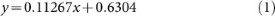where, y and x represent the center frequency and concentration of glucose, respectively. Therefore, the sensor exhibited a sensitivity of 112.67 MHz/mgmL−1 for the glucose-water solution. According to the optimization study and associated calibration plot (see Fig. 2 (d)), the detection limit of the assay for a signal-to-noise ratio (S/N) of 3 was calculated as 0.0621 μM of glucose in 5 μL of sample, as previously outlined31. The S-parameters for each sample were measured four times and the points deviated from the center frequency as shown by the error bars not overlapping for each concentration. Moreover, the maximum relative standard deviation (RSD) of 0.49% at the 0.5 mg/mL glucose level indicated by the error bar confirmed the excellent reproducibility of the proposed glucose biosensor for detecting glucose in deionized-water glucose solution. Fig. 2 (b) and (c) indicates the variations in the reflection coefficient (S11) and transmission coefficient (S21) of the resonator, respectively, for glucose samples of varying concentrations. S11 was maximized at −35 and −25 dB for glucose concentrations of 0.25 and 5 mg/mL, respectively. This behavior was expected, because the reflection coefficient of the resonator can be expressed by Equation (2), where, Pr (90) represents the reflected energy for normal incidence and corresponds to S11 for the present study and ε′ is negatively correlated with the glucose concentration32.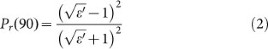However, the changes are not linear, because the reflected energy also reflects from the IDC electrodes and repeatedly impinges on the water-glucose solution in various ways. The bare resonator exhibited a transmission zero at 5.95 GHz, which constitutes one of the additional advantages of the resonator for glucose detection, because the position of the transmission zero and its amplitude level vary according the concentration of glucose in the solution. Fig. 2 (c) indicates that the position of the transmission zero shifted downward as a function of the glucose concentration, similar to the resonator center frequency. However, the magnitude of S21 varies non-linearly according to Equation (3), where Ptrans represents the energy transmitted via the resonator and corresponds to S21 for the present study. S21 was maximized at −13 and −18 dB for the 0.25 and 5 mg/mL glucose levels, respectively and thus varied in an opposite manner as S11. For the other glucose samples, the magnitude of S21 increased with the concentration in a non-linear manner.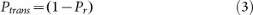The measured S-parameters of the resonators with five samples of human serum for the glucose level ranging from 1.48 to 2.28 mg/mL are shown in Fig. 2 (e). The nature of the center frequency shifts and the S11 variations are similar to these values in the glucose-water solutions. The regression analysis reveals a good linear correlation (r2 = 0.9998) between the glucose concentration and center frequency shift with the following linear regression equation: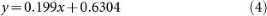Therefore, the sensor exhibited a sensitivity of 199 MHz/mgdL−1 for serum. According to the results of the optimization study and associated calibration plot with error bars (see Fig. 2 (f)), the detection limit of the assay for S/N = 3 was calculated as 0.033 μM of glucose in 5 μL of sample. Moreover, the maximum RSD of 0.61% at the glucose level of 2.28 mg/mL indicated by the error bar confirmed the excellent reproducibility of the proposed glucose biosensor for detecting glucose in serum. Supplementary Table S1 shows the values of the center frequencies of the proposed biosensor with relative standard deviations and other S-parameters for glucose samples of varying concentrations.

### Multi-dimensional detection using derived parameters

First, the propagation constant (γ) and impedance (Z) were derived from the measured S-parameters for the glucose samples of varying concentrations. The propagation constant spread from approximately 1.8 to 2.6 GHz, as depicted in Fig. 3 (a). This parameter promoted glucose sensing, because it exhibited a linear and positive correlation with the glucose concentration at frequencies ranging from approximately 2.2 to 2.4 GHz. Specifically, at 3.215 GHz, impedance precisely detected the level of glucose, because the impedance value increased with the glucose concentration at equal intervals, as indicated by Fig. 3 (b), except for the low level of glucose (0.25 mg/mL in this case). Additionally, resonance dips at different frequencies for various glucose levels were observed. The magnitude of this resonance dip was positively correlated with the glucose level. For further analysis, additional parameters, such as resistance (R), capacitance (C), inductance (L) and conductance (G), were obtained from the propagation constant and impedance using Equations (5) and (6)33.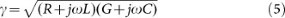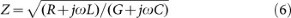The resistance increased with the increase in glucose concentration from approximately 2.56 to 2.75 GHz as shown in Fig. 3 (c). Resonance peaks, whose magnitude was positively correlated with glucose level, were also observed at different frequencies ranging from approximately 3 to 4.5 GHz. The inductance, which varied linearly and was positively correlated with the glucose concentration at 3.2 GHz, as shown in Fig. 3 (d), can be useful for glucose sensing. Furthermore, the inductance exhibited resonance peaks, whose magnitude was positively correlated with the glucose concentration. The resonator conductance exhibited clear variations with glucose concentrations from approximately 2.2 to 2.22 GHz, as illustrated in Fig. 3 (e) and also exhibited resonance peaks, whose magnitude increased with the glucose level. Fig. 3 (f) presents the capacitance of the biosensor chip for varying glucose concentrations and the capacitance was negatively correlated with the glucose concentrations for higher frequencies ranging from approximately 2 to 3 GHz. Resonance peaks were observed for frequencies ranging from approximately 1.3 to 1.8 GHz and their magnitude was positively correlated with the glucose level. Additionally, the frequencies of the capacitive resonance peaks were positively correlated with the glucose concentrations; therefore, the capacitance can also be useful for detecting glucose level. Supplementary Table S2 summarizes the measured values for the derived parameters for glucose samples of varying concentrations.

### Specificity of the glucose biosensor

The additional experiments were conducted using serum samples from three diabetic patients to determine the accuracy of the proposed glucose biosensor. These samples, which were tested at the hospital, contained different base glucose levels, as indicated in Table 1. To determine the glucose level using the proposed glucose biosensor, the S-parameters of the resonator were measured for 5 μL of each sample and the glucose concentration was determined from the center frequency using the calibrated Equation (4). The results, which are shown in Table 1, indicate that the glucose level in each serum sample was detected with significant accuracy. To study the effect of isomers, such as fructose or galactose, on the accuracy of glucose sensing, each serum sample was then supplemented with 0.1 mg/mL of fructose. Numerous molecules may potentially affect glucose sensing; however, the variation in the levels of these molecules is small in diabetic patients. Therefore, fructose was chosen for this study, because this molecule exhibits larger dynamic ranges dependent on various factors, such as the patient's diet. The recovery of the glucose level in the serum samples with fructose displayed negligible statistical variance, as indicated in Table 1, which confirmed the ability to sense glucose accurately in the presence of fructose. Thus, the proposed glucose biosensor retains high selectivity and fructose will likely have no impact on the precision or accuracy of glucose sensing.

### Reusable detection as a biosensor

To demonstrate the reusability of the proposed glucose biosensor, its resonating characteristics were observed for the following two conditions: the initial measurement of S-parameters for the bare resonator and the measured S-parameters for the resonator each time it was flushed to enable the measurement of a new glucose sample. Fig. 4, which compares the measured S-parameters for the mentioned conditions, indicates that the biosensor chip regained the original resonating characteristics after rinsing with PBS solution and drying. Additionally, the surface roughness of the sensing metal layer of the resonator was observed using AFM, which indicated that approximately similar roughness values were obtained prior to the measurement and after washing. Therefore, the proposed biosensor chip can be used to detect glucose levels repeatedly, which reduces the cost of the product over the long term.

Table 2 displays the comparisons among the proposed glucose biosensor and several previously reported glucose biosensors and shows that we developed a glucose biosensor with the lowest limit of detection. Therefore, the proposed glucose biosensor is more suitable for the early-stage detection of diabetes. The accuracy of the proposed glucose biosensor and biosensor reported in reference 23 was improved by multidimensional detection. However, the proposed glucose biosensor exhibited significantly higher sensitivity for glucose detection, as indicated by the marked increase in shift of the center frequency for lower variation in glucose concentration. Additionally, the proposed glucose biosensor has a comparable response time with all of the reported glucose biosensors and has an excellent reproducibility for glucose detection. Moreover, this work developed a more cost-effective and reusable biosensor.

## Discussion

In this work, rapid, sensitive and reusable glucose detection by an IPD chip on a GaAs substrate was developed for the mediator-free detection of glucose. The IDC between the two divisions of an intertwined spiral inductor strongly couples the electromagnetic energy, as illustrated by the simulated current density at the center frequency in Fig. 1 (b). Therefore, the IDC can transform even a small change in the permittivity caused by changing the glucose concentration into effective capacitance variations. Accordingly, the IDC transforms the shift in the center frequency and other characteristics of the resonator. Fig. 5 (d) depicts the scanning electron microscopy (SEM) image of the IDC with a schematic view of water and monosaccharide molecules. The electric modeling of a portion of the IDC-bearing glucose sample is illustrated in Fig. 5 (e). Fig. 5 (f) depicts the detailed layer information of the IPD fabrication process of the proposed resonator. The equivalent admittance of the capacitor, whose equivalent circuit is depicted in Fig. 5 (e), is expressed as follows: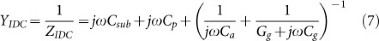where, ZIDC is the equivalent impedance of the IDC, Cp and Csub represent the capacitive effects due to the direct flux and flux via the substrate, respectively, from the source to ground electrode, Ca represents the capacitive effect due to flux via air and Cg and Gg represent the capacitance and conductance due to flux via the glucose sample, respectively. The net capacitance of the IDC with the glucose sample can be expressed as follows: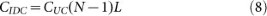where, the unit cell capacitance CUC can be expressed as follows: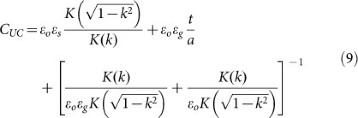where k = a/b and K(k) is the elliptic integral of the first kind34, N is the number of unit cells, L is the length of the coupled electrode, ε0 is the free space permittivity, εs is the GaAs substrate permittivity and εg is the permittivity of the glucose sample.

According to the Debye dispersion model, the dielectric constant (εg) and loss factor (ε″g) of the glucose sample can be expressed as a complex quantity as follows: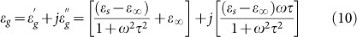The above equation is only an approximation and is used to study the effect of the sample glucose concentration on permittivity. Monosaccharide molecules (C6H12O6) contain a higher number of –OH groups that form more –H bonds when present as a monomer in water, resulting in less available water to interact with the AC field. Therefore, the dielectric constant of a water-glucose solution is lower than water. Furthermore, glucose has a dipole moment of 3.8 and a molecular weight of 180.2 kDa. Thus, the glucose molecule is heavier than the water molecule, which contributes to the dielectric mechanism of aqueous glucose in the following ways: 1) the viscous effect is more pronounced due to its large size, resulting in a difficult rotation with the AC field and (2) its relatively large dipole moment does not provide the molecule with the needed compactness to facilitate reorientation with the AC field. Therefore, the viscous effect increases as the concentration of the glucose solution increases, resulting in increased relaxation times and correspondingly decreased dielectric constants and increased loss factor according to Equation (10)35,36. As a consequence, the capacitance of the sensing capacitor in this study is expected to be maximized and minimized when the glucose level in the glucose sample is minimized and maximized at 0.25 and 5 mg/mL (for the glucose-water solution) and, 1.48 and 2.28 mg/mL (for serum), respectively.

The proposed RF resonator-based biosensor chip thus enabled clear and sensitive detection of glucose within the diabetic range based on the shift in center frequency, which is guided by effective changes in inductance and capacitance due to the complex interaction between the glucose sample and chip. The multiple parameters derived from the measured S-parameters resulted in unidirectional sensing and thus increased the precision of detection. Specifically, the impedance was highly sensitive and exhibited a linear and positive correlation with glucose concentration. Additionally, the proposed glucose biosensor is reusable and has advantages, such as a short assay time and a micromolar detection limit (0.0621 μM for water-glucose solution and 0.033 μM for serum). Thus, this approach was demonstrated to be a successful electromagnetic spectroscopy-based biosensor and can potentially be applied in point-of-care testing for diabetes monitoring.

## Methods

### Fabrication of the RF resonator

The RF resonator was fabricated using an integrated passive device (IPD) process and the focused ion beam (FIB) image of the fabricated resonator is shown in Fig. 1 (d)37,38. To fabricate the biosensor resonator, silicon nitride (SiNx, 200 nm) was first deposited over a gallium arsenide (GaAs, 400 μm) substrate as a passivation layer using plasma-enhanced chemical vapor deposition (PECVD). A 2-μm-thick Au metal layer, followed by a 20/80 nm Ti/Au seed metal via the RF sputtering process, were then formed by electroplating and, were used as the metal lines for the IDC and coils for the spiral inductor. The passivation layer enhances the adhesion between the substrate and first metal layer. Again, a seed metal layer was formed as previously mentioned, followed by a second passivation layer of 200 nm of SiNx deposited by PECVD to prevent any possible shortage between the first and second metal layers. An air-bridge photo process, was then performed prior to the Au (3 μm) top metal definition and plating process, by which air-bridge interconnections were formed at broken coil paths around a metal beeline for the inductor. After the electroplating process, the air-bridge mask was stripped and the reactive ion etching (RIE) of the Ti/Au seed metal was performed.

### Sample preparation

The following two classes of glucose samples were prepared: a glucose stock solution that consisted of a mixture of deionized water (Merck Millipore, Billerica, Massachusetts, USA) and D-glucose powder (SIGMA, life science, GC) and serum prepared from blood that was extracted from healthy human subjects. The glucose/water samples were prepared at the following 11 different concentrations: 0.25, 0.5, 1, 1.5, 2, 2.5, 3, 3.5, 4, 4.5 and 5 mg/mL. The base glucose level of the serum obtained by centrifuging the blood at 3000 rpm for 12 min was 1.48 mg/mL. The 5 different concentrations of the prepared serum were 1.48, 1.68, 1.88, 2.08 and 2.28 mg/mL. In this study, the solutions of deionized-water and D-glucose powder, sera from human blood and sera with supplemented fructose were prepared at ROS Medical Research Center, Department of Biochemistry and Molecular Biology, Kyung-Hee University, Seoul, South Korea and all experimental protocol were approved by the ethical committee of Kyung-Hee University, Seoul, South Korea. Informed written consent was obtained from all subjects before collecting their blood samples for glucose testing and all human procedures were performed in accordance with the guidelines and regulations of South Korea's Bioethics and Biosafety Act (2005). The experiments for studying the glucose-sensing performance of the biosensor were conducted at Kwangwoon University, Seoul, South Korea and all experimental protocol were approved by Fusion Technology Center, Kwangwoon University, South Korea.

### Measurements

To perform the electrical measurements of the resonator using an Agilent 8510C vector network analyzer (VNA) as illustrated in Fig. 5 (a), the fabricated resonator was wire-bonded with 50 Ω transmission lines, as depicted in Fig. 5 (b). Five microliters of samples was placed on the resonator surface using a Finnpipette (5–50 μL, Thermo Electron Corporation). The S-parameters were measured over a frequency range of 0.1 to 12 GHz. To maintain the sample at a constant temperature, all of the samples were equilibrated to room temperature prior to testing. To measure the effectiveness of this equilibration, the temperatures of each individual sample were measured with a thermocouple probe immediately prior to the electrical measurements on each particular sample. The measured temperatures of the individual samples ranged from 19.3 to 20.7°C. After the electrical measurement for a sample, the resonator surface was flushed several times using deionized water (for glucose-water solution) and phosphate-buffered saline (PBS) solution (for serum) to remove the glucose sample prior to measuring the new sample.